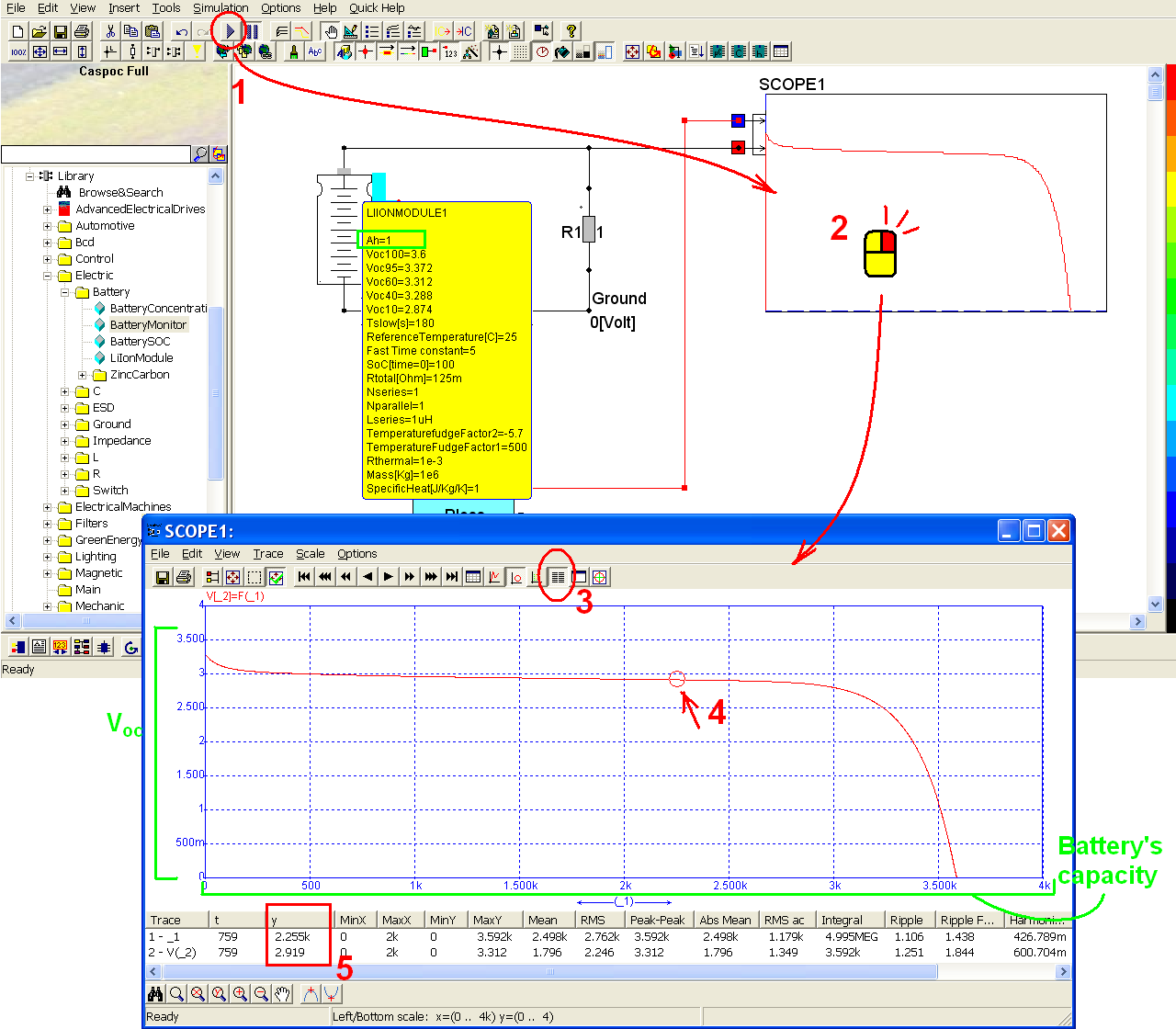## Battery

Lithium-ion battery is widely used because of its high capacity and voltage. In this tutorial, we will build a simple example to show the characteristics of a lithium-ion battery and explain its available parameters. The circuit we�re going to build is shown as below: A Li-ion battery and its electrical load. The capacity of a battery is calculated in ampere-hour (Ah) namely the product of the current and the discharge time. The higher the electrical load, the smaller current it is. Since the capacity of a battery is fixed, a smaller current results in a longer discharge time.

Another problem for a battery is the environment temperature. In Caspoc, the Li-Ion battery also provides users an extension for a thermal circuit. Because of the temperature variation, the characteristic curve changes according to the fudge factors.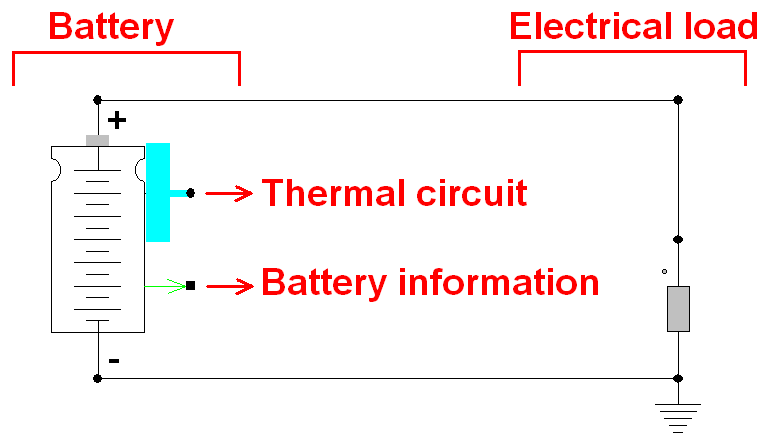First, take the Li-Ion battery from the component list. Left-click Components/Library/Electric/Battery/LiIonModule (step 1) and release the mouse. The Li-Ion module follows the cursor and left-click to put it on the workscreen (step 2). Right-click the Li-Ion module to see its parameters. In the pop-window, we can see the parameters as below (step 3):

• Ah (battery capacity: ampere-hour) = 1 (maximum charge of the battery)
• Fast Time constant = 5 second (The time constant related to the SEI grow of the electric double layer. It is much smaller than slow time constant and mostly in the order of several seconds.)
• Lseries = 1uH (series inductance of the battery due to the wiring inside the battery)
• Mass = 1e6 kg (the mass of the battery)
• Nparallel = 1 (number of cells connected in parallel inside the battery module) (Notices that all the parameters in other fields are specified for a single cell.)
• Nseries = 1 (number of cells connected in series inside the battery module) (Notices that all the parameters in other fields are specified for a single cell.)
• Reference Temperature = 25 �C (These Voc values and Rtotal in this dialogue block are specified in datasheet at this reference temperature.)
• Rthermal = 1e-3 K/W (thermal resistance between battery cells and the thermal connection of the battery module)
• Rtotal = 125m ohm (total series resistance of this battery module)
• SoC(time=0) = 100% (initial state of charge of this battery) (100 stands for a fully loaded battery or any other value between 0 and 100 for a partially discharged battery)
• SpecificHeat = 1 J/Kg/K (the self heating of the battery) (a value close to 1 is averagely a default value for many battery materials)
• TemperatureFudgeFactor1 = 500 (temperature fudge factor for making the Voc table relates to the decrease of state of charge)
• TemperatureFudgeFactor2 = -5.7 (temperature fudge factor for making the Voc table relates to the decrease of series resistance)
• Tslow = 180 seconds (Slow time constant related to the mass transport inside the battery. Mostly in the order of several tens of seconds to minutes.)
• Voc10 = 2.874 volts (Voc while SoC = 10%)
• Voc100 = 3.6 (Voc while SoC = 100% for no load current)
• Voc40 = 3.288 (Voc while SoC = 40% for no load current)
• Voc60 = 3.312 (Voc while SoC = 60% for no load current)
• Voc95 = 3.372 (Voc while SoC = 95% for no load current)

Click OK to save the setting if needed (step 4).

Here we don�t connect any thermal circuit. If the thermal circuit is not connected, make sure the parameter for the mass is set to a large value in order to keep a constant initial battery temperature. If connected to a thermal circuit, correctly specify the parameters Mass, specificHeat and Rthermal inside the battery.Left-click Components/Circuit/RLC/R (step 1) and release the mouse. The resistor follows the cursor and right-click to change its direction (step 2). Until the anode is upward, left-click on the workscreen to put the resistor in the right side of the Li-Ion module (step 3). Right-click on the resistor to modify the parameter. In the pop-window, enter value = 1 (step 4) and click OK to save the setting (step 5).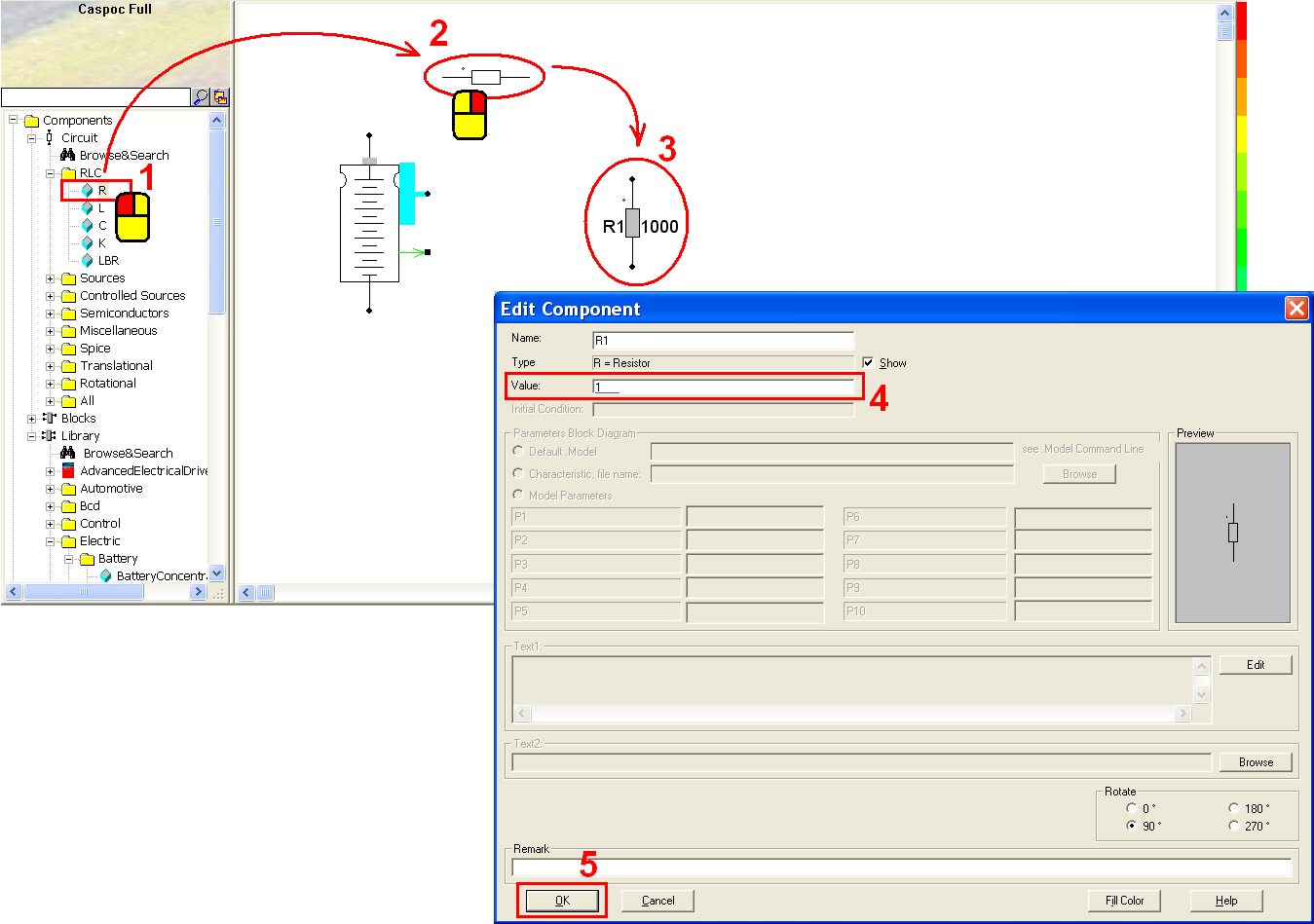Connect the Li-Ion module and the resistor with the following configuration (step 1). Currently we have not set an electric ground. Right-click the cathode of the resistor to add a ground label (step 2). In the pop-window, enter label = Ground (step 3) and click OK to save the setting (step 4).Then we want to get the voltage-SoC characteristic curve of the Li-Ion battery as below, that is, take the SoC value from the battery as the X-axis and the voltage value through the resistor as the Y-axis.To read the SoC value of the battery, we can directly connect the SoC output to a scope. Or use a battery monitor which provides the information which can be calculated from the SoC. Left-click Component/Library/Electric/Battery/BatteryMonitor (step 1) and release the mouse. Left-click to put this battery monitor below the circuit we built (step 2). Connect the battery monitor and the Li-Ion module with the following configuration (step 3).Click the scope iconin the experience bar (step 1) to put a scope on the workscreen (step 2). Left-click the right-bottom corner of the scope and hold down the mouse to enlarge this scope (step 3).SoC and SoD of the battery monitor stand for state of charge and discharge (from 0 to 100%). The sum of SoC and SoD equals to 100%. To get a decreasing SoC as the X-axis, we can use an increasing SoD. Connect the SoD output of the battery monitor to the first blue input trace of the scope (step 1). Connect the anode of the resistor to the second red input trace of the scope (step 2). Right-click on the scope (step 3) to change the data display. In the pop-window, click View/XY Writer (step 4) and then close it.Click the short-cut of simulation parameter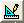(step 1). In the pop-window, select Trapezoidal for the Numerical Integration Method (step 2), Tscreen = 2k, dt = 1 (step 3) and then click OK to save the setting (step 4).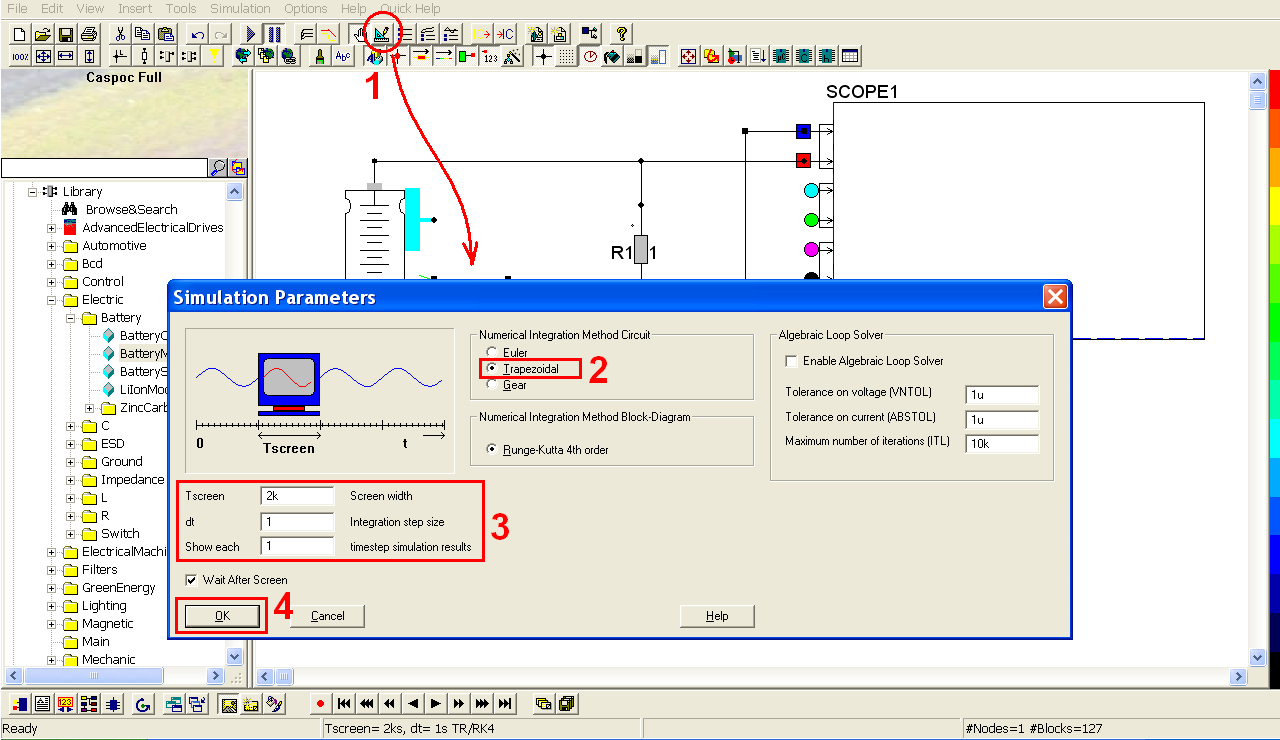Click the short-cut of start simulation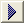(step 1). Right-click on the scope (step 2) to see the details of the simulation result. Click the shortcut of listbox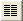(step 3) to enable the numeric display of simulation result. Click the Right or Left key to find the value change as time goes by (step 4 and 5).

Here we can see that the voltage start from Voc100 because of the initial SoC is 100%. Then we can see the Voc values of the battery module are a bit higher than the result because of the electrical load.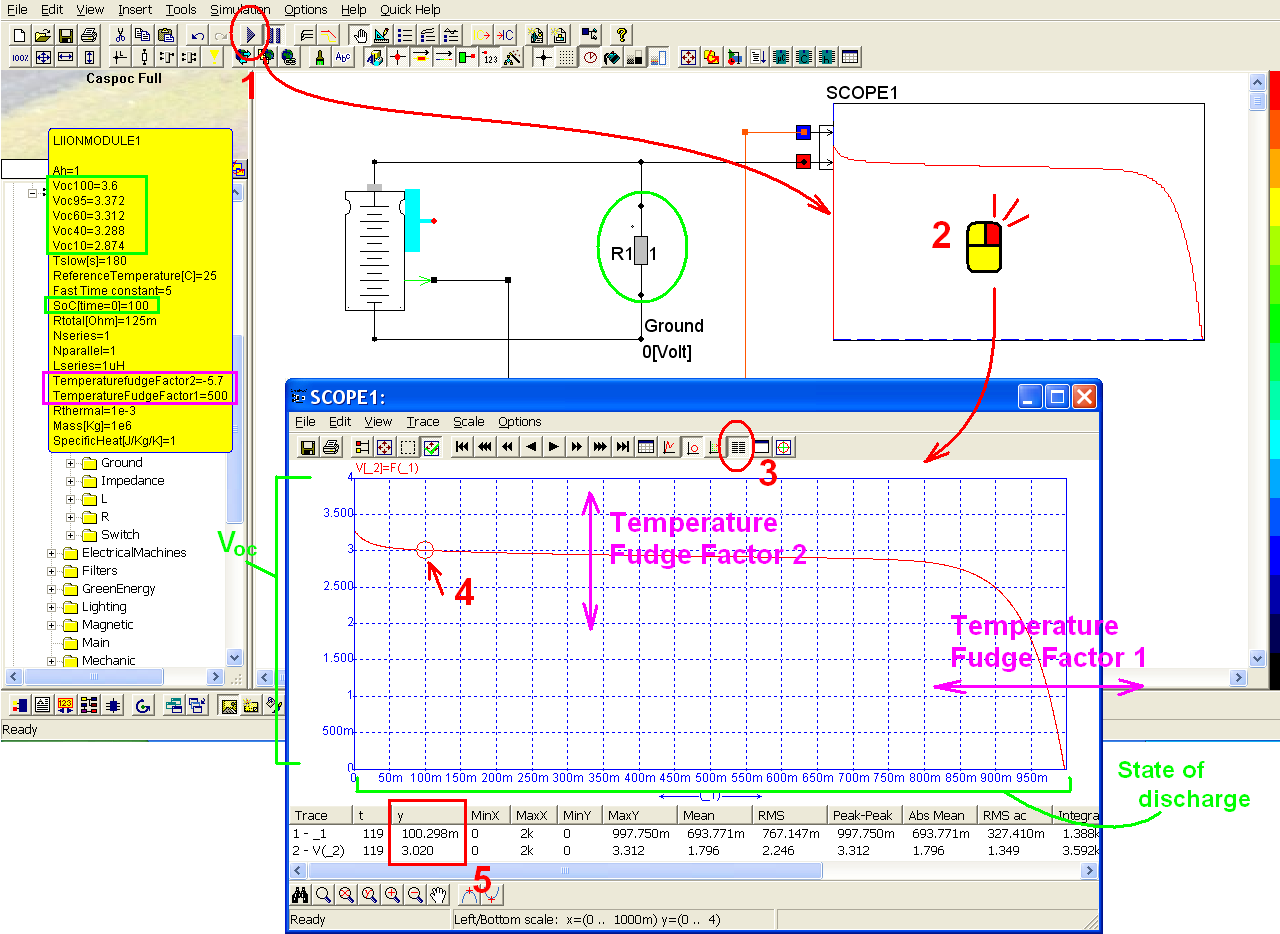The resistance of the electrical load affects the characteristic curve of the battery module and also the discharge time of the battery. You can try to change the value of the resistor and see how it impacts the simulation result. The animation of the battery will be also shown according the state of discharge, as below.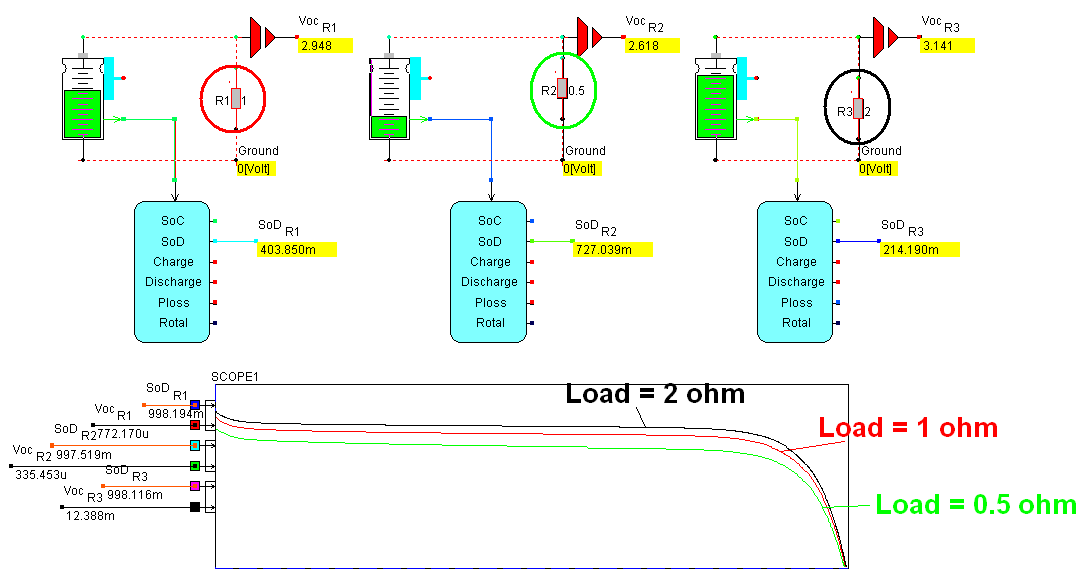Then we try to change the X-axis from the SoC to the discharge value which stands for the discharge ampere-hour of the battery. As well as SoD and SoC, the sum of the charge and discharge shall equal to the battery capacity (the parameter Ah of the battery). If the battery is charged from 0% (state of charge), it will be able to reach the maximum capacity of the battery (in Ampere-hour). If the battery is discharged from 100% (state of charge), it will be able to discharge the total amount of the battery capacity too.

Select the connection between the battery monitor and the scope (step 1). Click Delete key to delete this connection (step 2).Connect the discharge output of the battery monitor to the first blue input trace of scope.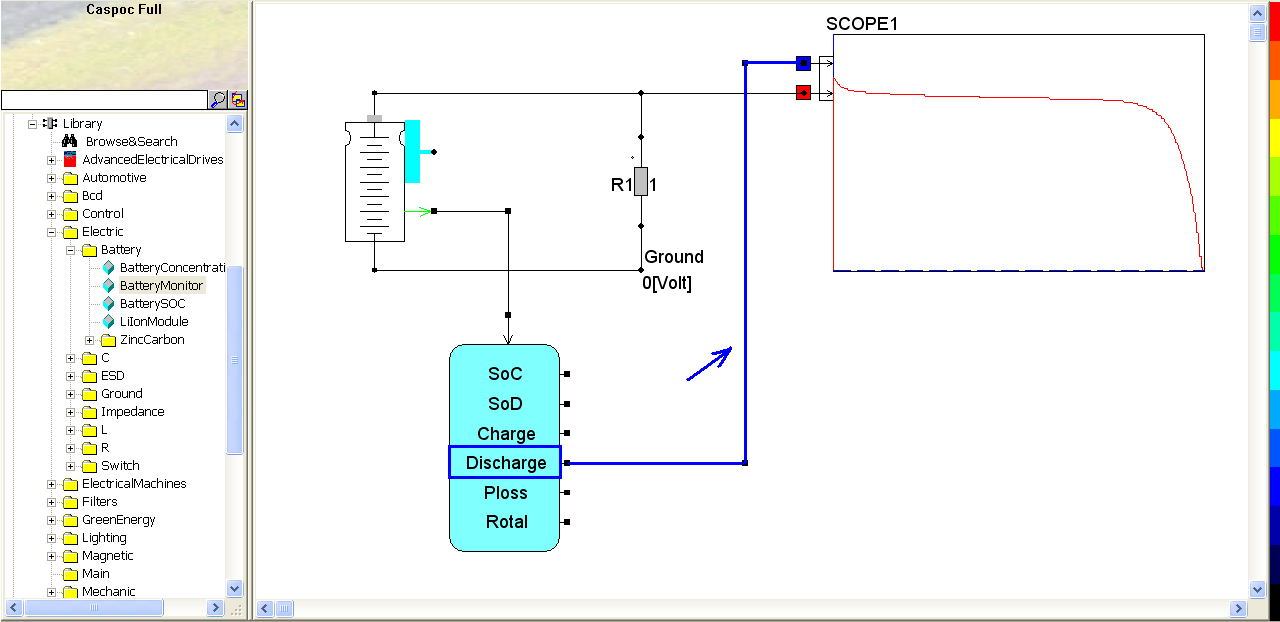Click the short-cut of start simulation(step 1). Right-click on the third scope (step 2) to see the details of the simulation result. In the pop-window, click the shortcut of listbox(step 3) to enable the numeric display of simulation result. Click Right or Left key to find the value change as time goes by (step 4). Since we change the SoC to discharge. The discharge coulombs roughly equal to the product of the current through the resistor and the time: Q = I*time = 2.929/1 * 759 ~2255 C (step5). The capacity of the battery is what we set in the parameters namely 1 Ah = 3600 C.